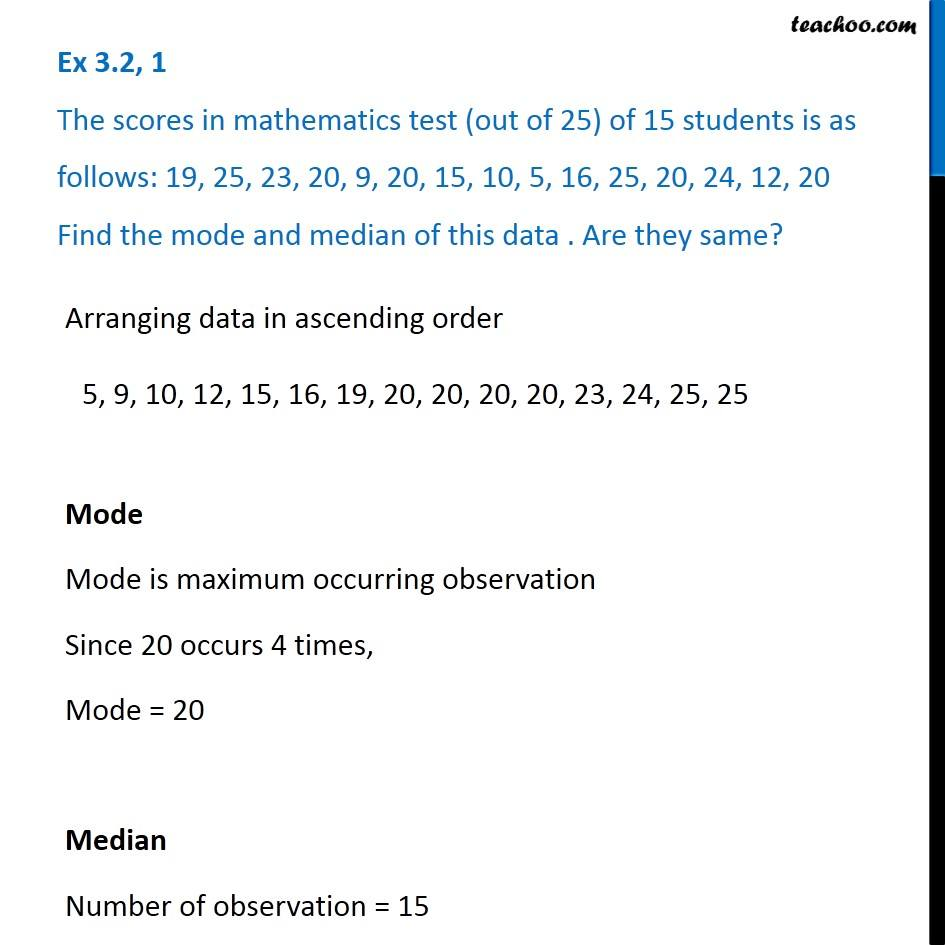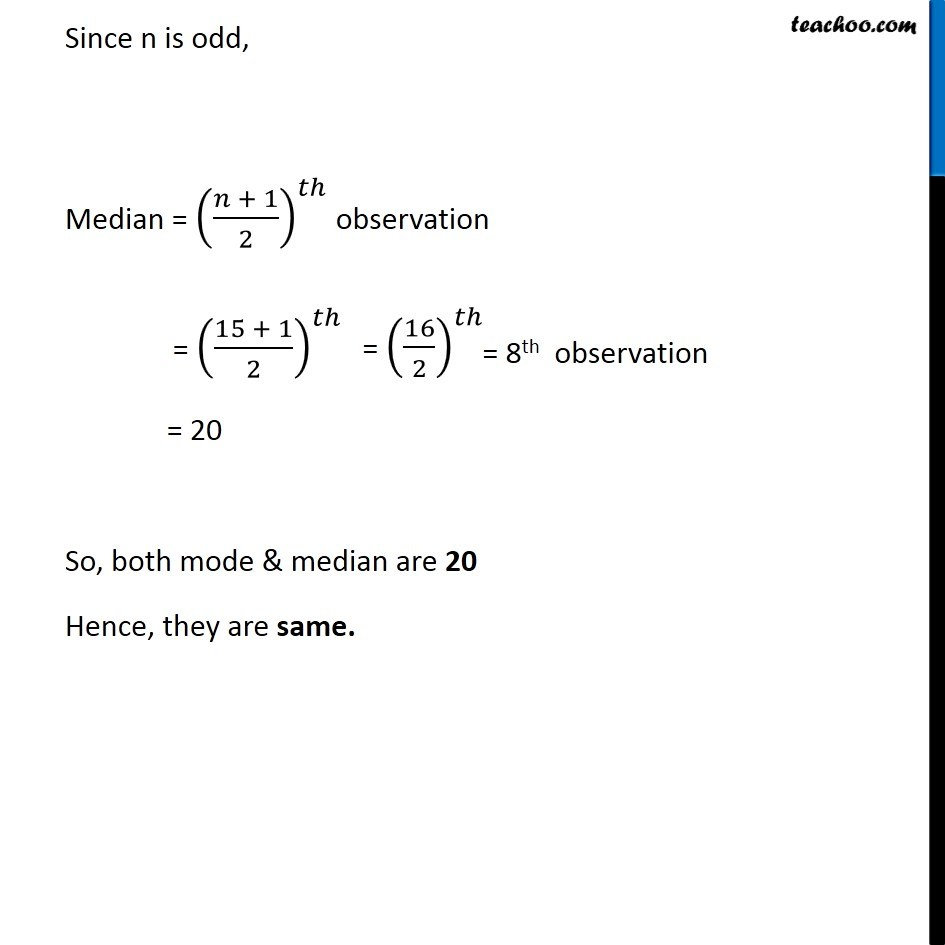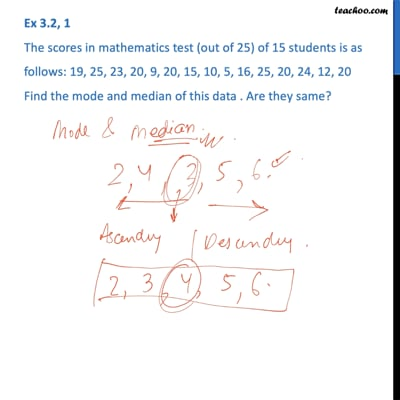Ex 3.2

Chapter 3 Class 7 Data Handling
Serial order wiseThis video is only available for Teachoo black users

### Transcript

Ex 3.2, 1 The scores in mathematics test (out of 25) of 15 students is as follows: 19, 25, 23, 20, 9, 20, 15, 10, 5, 16, 25, 20, 24, 12, 20 Find the mode and median of this data . Are they same? Arranging data in ascending order Mode Mode is maximum occurring observation Since 20 occurs 4 times, Mode = 20 Median Number of observation = 15 Since n is odd, Median = ((𝑛 + 1)/2)^𝑡ℎ observation = ((15 + 1)/2)^𝑡ℎ = 20 So, both mode & median are 20 Hence, they are same.